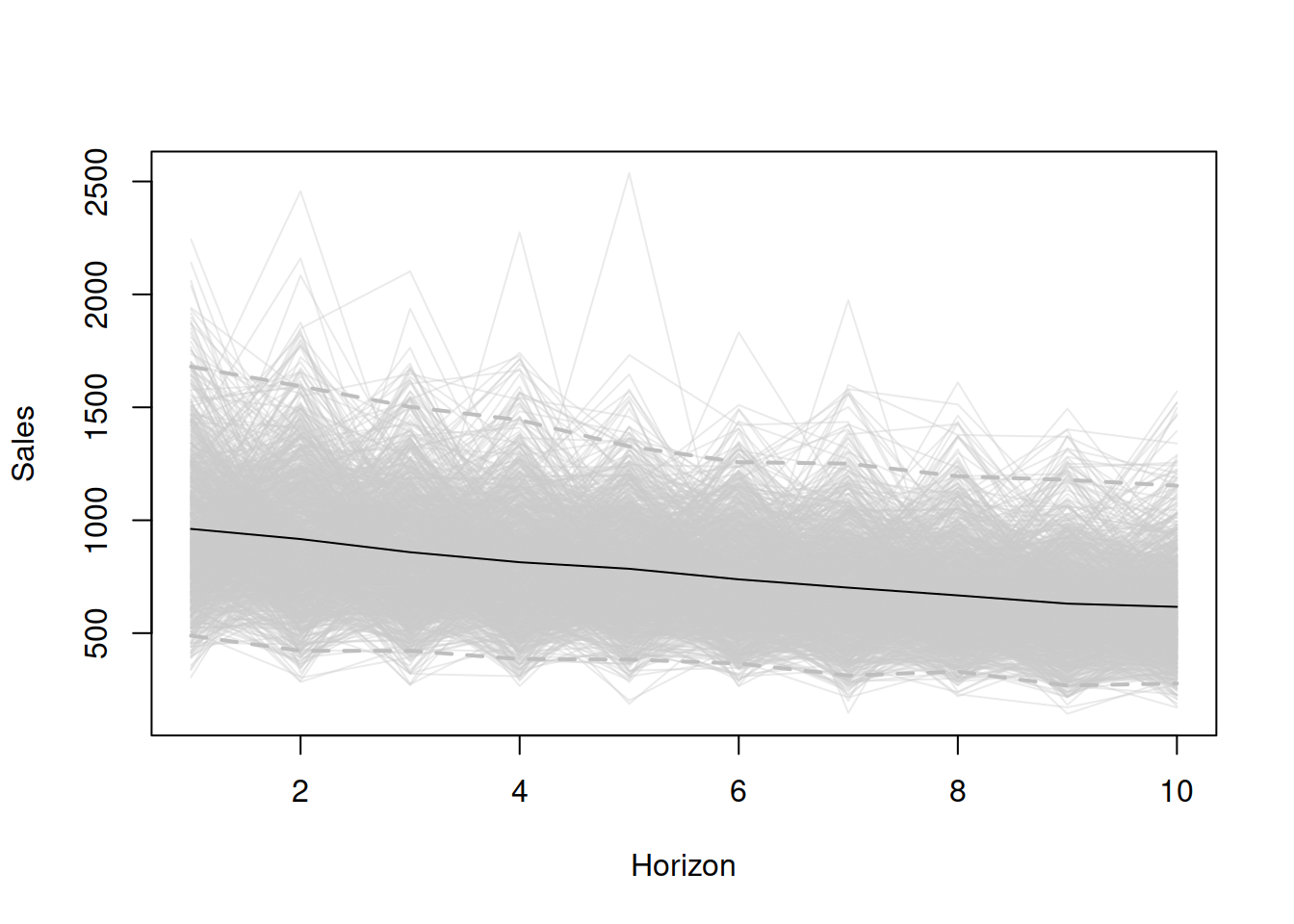This book is in Open Review. I want your feedback to make the book better for you and other readers. To add your annotation, select some text and then click the on the pop-up menu. To see the annotations of others, click the button in the upper right hand corner of the page

## 17.1 Conditional expectations

As discussed in sections 5.3 and 6.3, the conditional h step expectations are in general available only for the pure additive models. In the cases of pure multiplicative models, the point forecasts would correspond to conditional expectations only for $$h \leq m$$ for seasonal models and $$h=1$$ for models with trend. The one exception is ETS(M,N,N) model, where the point forecast corresponds to the conditional expectation for any horizon. When it comes to the mixed models (Section 7.2), the situation would depend on the specific model, but in general the same logic as for the pure multiplicative ones applies. In this case, the conditional expectations need to be obtained via other means. This is when the simulations come into play.

### 17.1.1 Simulating demand trajectories

The general idea of this approach is to use the estimated parameters, last obtained state vector (level, trend, seasonal, ARIMA components etc) and the estimate of the scale of distribution in order to generate the possible paths of the data. The simulation itself is done in several steps after obtaining parameters, states and scale:

1. Generate $$n \times h$$ (where $$n$$ is the number of time series to produce) random variables for the error term, $$\epsilon_t$$ or $$1+\epsilon_t$$ - depending on the type of error and assumed distribution in the model (the latter was discussed in section 5.5 and 6.5);
2. Generate actual values from $$n$$ models with the provided state vector, transition, measurement and persistence matrices and the generated error terms fo the next $$h$$ observations. In a general case, this is done using the model (7.1);
3. Take expectations for each horizon from 1 to $$h$$.

A thing to note that in case of multiplicative trend or multiplicative seasonality, it makes sense to take trimmed mean instead of the basic arithmetic one. The reason for this is because the models with these components might exhibit explosive behaviour and thus the expectation might become unrealistic. I suggest using 1% trimming, although this does not have any scientific merit and is only based on my personal expertise.

The simulation-based approach is universal, no matter what model is used and can be applied to any ETS, ARIMA, regression model or their combination (including dynamic ETSX, intermittent demand and multiple frequency models). Furthermore, instead of taking expectations on step 3, one can take geometric means, medians or any desired quantile. This is discussed in some detail later in Section 17.2.2.

The main issue with this approach is that the conditional expectation and any other statistic calculated based on this, will differ with every new simulation run. If $$n$$ is small then these values will be less stable (vary more with the new runs). But they will reach some asymptotic values with the increase of the value of $$n$$, staying random nonetheless. However, this is in a way a good thing, because this randomness reflects the uncertain nature of these statistics in sample. Another limitation is the computational time and memory usage: the more iterations we want to produce, the more calculations and more memory will need to be done. Luckily, time complexity in this situation should be linear: $$O(h \times n)$$.

### 17.1.2 Explanatory variables

If the model contains explanatory variables, then the h steps ahead conditional expectations should rely us them in the calculation. The main challenge in this situation is that in some cases the future values might not be known. Both cases have been discussed in the Section 10.2. Practically speaking, if the user provide the holdout sample values of explanatory variables, then forecast.adam() method will use them in forecasting. If they are not provided, then the function will produce forecasts for each of the explanatory variables via adam() function and use the conditional h steps ahead expectations in forecasting.

### 17.1.3 Demonstration in R

In order to demonstrate how the approach works, we consider an artificial case of ETS(M,M,N) model with $$l_t=1000$$, $$b_t=0.95$$, $$\alpha=0.1$$, $$\beta=0.01$$, and Gamma distribution for error term with scale $$s=0.05$$. We generate 1000 scenarios from this model for the horizon of $$h=10$$ using sim.es() function from smooth package:

nsim <- 1000
h <- 10
s <- 0.1
initial <- c(1000,0.95)
persistence <- c(0.1,0.01)
y <- sim.es("MMN", obs=h, nsim=nsim, persistence=persistence,
initial=initial, randomizer="rgamma",
shape=1/s, scale=s)

After running the code above, we will obtain an object that contains several variables, including y$data with all the 1000 possible future trajectories. We can plot them to get an impression of what we are dealing with (see Figure 17.1). plot(y$data[,1], ylab="Sales", ylim=range(y$data), col=rgb(0.8,0.8,0.8,0.2), xlab="Horizon") for(i in 2:nsim){ lines(y$data[,i], col=rgb(0.8,0.8,0.8,0.2))
}
lines(apply(y$data,1,mean)) lines(apply(y$data,1,quantile,0.025),
col="grey", lwd=2, lty=2)
lines(apply(y\$data,1,quantile,0.975),
col="grey", lwd=2, lty=2)Figure 17.1: Data generated from 1000 ETS(M,M,N) models.

Based on the plot in Figure 17.1, we can see what the conditional h steps ahead expectation will be (black line) and what the 95% prediction interval will be for the data based on the ETS(M,M,N) model with the parameters mentioned above.